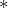Time-to-depth conversion and seismic velocity estimation using time-migration velocityNext: Introduction Up: Reproducible Documents

# Time-to-depth conversion and seismic velocity estimation using time-migration velocity

Maria Cameron, Sergey Fomel, and James SethianDepartment of Mathematics,
Courant Institute of Mathematical Science,
New York University,
251 Mercer Street, New York, NY 10012Bureau of Economic Geology,
John A. and Katherine G. Jackson School of Geosciences
The University of Texas at Austin
University Station, Box X
Austin, TX 78713-8972Department of Mathematics,
University of California, Berkeley,
Berkeley, CA, 94720

### Abstract:

The objective of this work is to build an efficient algorithm (a) to estimate seismic velocity from time-migration velocity, and (b) to convert time-migrated images to depth. We establish theoretical relations between the time-migration velocity and the seismic velocity in 2-D and 3-D using paraxial ray tracing theory. The relation in 2-D implies that the conventional Dix velocity is the ratio of the interval seismic velocity and the geometrical spreading of the image rays. We formulate an inverse problem of finding seismic velocity from the Dix velocity and develop a numerical procedure for solving it. This procedure consists of two steps: (1) computation of the geometrical spreading of the image rays and the true seismic velocity in the time-domain coordinates from the Dix velocity; (2) conversion of the true seismic velocity from the time domain to the depth domain and computation of the transition matrices from time-domain coordinates to depth.

For step 1, we derive a partial differential equation (PDE) in 2-D and 3-D relating the Dix velocity and the geometrical spreading of the image rays to be found. This is a nonlinear elliptic PDE. The physical setting allows us to pose a Cauchy problem for it. This problem is ill-posed. However we are able to solve it numerically in two ways on the required interval of time. One way is a finite difference scheme inspired by the Lax-Friedrichs method. The second way is a spectral Chebyshev method. For step 2, we develop an efficient Dijkstra-like solver motivated by Sethian's Fast Marching Method.

We test our numerical procedures on a synthetic data example and apply them to a field data example. We demonstrate that our algorithms give significantly more accurate estimate of the seismic velocity than the conventional Dix inversion. Our velocity estimate can be used as a reasonable first guess in building velocity models for depth imaging.Time-to-depth conversion and seismic velocity estimation using time-migration velocityNext: Introduction Up: Reproducible Documents

2013-03-02